# JEE Main 2021 March 16 – Shift 2 Physics Question Paper with Solutions

JEE Main 2021 March 16th Shift 2 Physics Question Paper is provided here. Students can have a glance at the question paper and get a better understanding of the question types, difficulty level, marking scheme and the overall nature of the question paper. JEE Main 2021 Physics March 16, Shift 2 paper has been solved by our subject experts and they have provided solutions in detailed manner. This study material will help the students to prepare effectively for the exam and attempt maximum questions in the stipulated time by increasing their speed and accuracy.

### JEE Main 2021 March 16th Shift 2 Physics Question Paper

Section A

Question 1. A mosquito is moving with a velocity $\vec{v} = 0.5t^{2}\hat{i} + 3t\hat{j} + 9\hat{k}$ m/s and accelerating in uniform conditions. What will be the direction of the mosquito after 2s?

1. a. tan-1 (⅔)  from x - axis
2. b. tan-1 (5/2) from x - axis
3. c. tan-1 (⅔)  from y - axis
4. d. tan-1 (5/2) from y - axis

Solution:

Given,

$\vec{v} = 0.5t^{2}\hat{i} + 3t\hat{j} + 9\hat{k}$

$\vec{v}_{t=2} = {2}\hat{i} + 6\hat{j} + 9\hat{k}$

$\left | \vec{v} \right | = \sqrt{2^{2}+6^{2}+9^{2}} = 11 m/s$

∴ angle made by direction of motion of mosquito will be –

cos-1 (2/11) from x - axis = tan-1 (√117/2)

cos-1 (6/11) from y - axis = tan-1 (√85/6)

cos-1 (9/11) from z - axis = tan-1 √40/9

No option is matching

Question 2. Statement I: A cyclist is moving on an unbanked road with a speed of 7 kmh-1 and takes a sharp circular turn along a path of radius of 2m without reducing the speed. The static friction coefficient is 0.2. The cyclist will not slip and pass the curve.

(g = 9.8 m/s2)

Statement-II: If the road is banked at an angle of 45°, cyclists can cross the curve of 2m radius with the speed of 18.5 kmh-1 without slipping.

In the light of the above statements, choose the correct answer from the options given below:

1. a. Both statement I and statement II are false
2. b. Both statement I and statement II are true
3. c. Statement I is correct and statement II is incorrect
4. d. Statement I is incorrect and statement II is correct

Solution:

On a horizontal ground,

$v_{max} = \sqrt{\mu Rg} = \sqrt{0.2 \times 2 \times 9.8 } = 1.97 m/s = 1.97 \times\frac{18}{5}$

= 7.12 km/hr= 7.2 km/hr > given velocity.

Hence it can turn safely.

Statement-2

$v_{max} = \sqrt{gr\left ( \frac{tan\Theta+\mu }{1-\mu tan\Theta } \right )} = \left ( \sqrt{2\times9.8 \times \frac{1.2}{0.8}} \right )\times \frac{18}{5} = 19.5km/hr$

$v_{max} = \sqrt{rg\left ( \frac{tan\Theta-\mu }{1+\mu tan\Theta } \right )} = \left ( \sqrt{2\times9.8 \times \frac{0.8}{1.2}} \right )\times \frac{18}{5} = 12.01km/hr$

Since given velocity is between vmax and vmin, cyclists can safely turn.

Question 3. Calculate the time interval between 33% decay and 67%decay if half-life of a substance is 20 minutes:

1. a. 40 minutes
2. b. 20 minutes
3. c. 60 minutes
4. d. 13 minutes

Solution:

Nt = N0e-λt

⇒ ln (0.67 / No) = –λt1 ...(1)

and ln (0.37 / No) = –λt2 ...(2)

Equation (1) – Equation (2)

⇒ λ(t2 – t1) = ln (0.67 / 0.37) = ln2

⇒ t2 – t1 = ln2 / λ = t1/2

= 20 min.

Question 4. A large block of wood of mass M = 5.99 kg is hanging from two long massless cords. A bullet of mass m = 10 g is fired into the block and gets embedded in it. The (block + bullet) then swing upwards, their centre of mass rising a vertical distance h = 9.8 cm before the (block + bullet) pendulum comes momentarily to rest at the end of its arc. The speed of the bullet just before the collision is: (take g = 9.8 ms-2)1. a. 831.4 m/s
2. b. 841.4 m/s
3. c. 811.4 m/s
4. d. 821.4 m/s

Solution:

Pi = 0.01 × u + 0 = Pf = 6 × v

v = 0.01u / 6 m/s

using energy conservation

½ × 6 × v2 = 6 × g × h

= ½ × (0.01u / 6)2 = 9.8 × 9.8 × 10-2

= u = 9.8 × 6 √2 × 10

= 831.4 m/s

Question 5. What will be the nature of the flow of water from a circular tap, when its flow rate increased from 0.18 L/min to 0.48 L/min? The radius of the tap and viscosity of water is 0.5 cm and 10-3 Pa s, respectively. (Density of water: 103 kg/m3)

4. d. Remains turbulent flow

Solution:

The nature of flow is determined by Reynold’s no.

$Re=\frac{pvD}{\eta }$

if Re < 1000 → flow is steady

1000 < Re < 2000 → flow becomes unsteady

Re > 2000 → flow is turbulent

(Re)initial = $10^{3}\times \frac{0.18\times 10^{-3}}{\pi \times (0.5\times 10^{-2})^{2}\times 60}\times \frac{1\times 10^{-2}}{10^{-3}}=382.16$

(Re)final = $10^{3}\times \frac{0.48\times 10^{-3}}{\pi \times (0.5\times 10^{-2})^{2}\times 60}\times \frac{1\times 10^{-2}}{10^{-3}}=1019.09$

Question 6. The refractive index of a converging lens is 1.4. What will be the focal length of this lens if it is placed in a medium of the same refractive index? Assume the radii of curvature of the faces of the lens are R1 and R2 respectively.

1. a. Zero
2. b. $\frac{R_{1}R_{2}}{R_{1}-R_{2}}$
3. c. Infinite
4. d. 1

Solution:

$\frac{1}{f}=\left [ \frac{n_{2}}{n_{1}}-1 \right ]\left [ \frac{1}{R_{1}}-\frac{1}{R_{2}} \right ]$

1/ f = 0

f = infinite.

Question 7. For the given circuit, comment on the type of transformer used.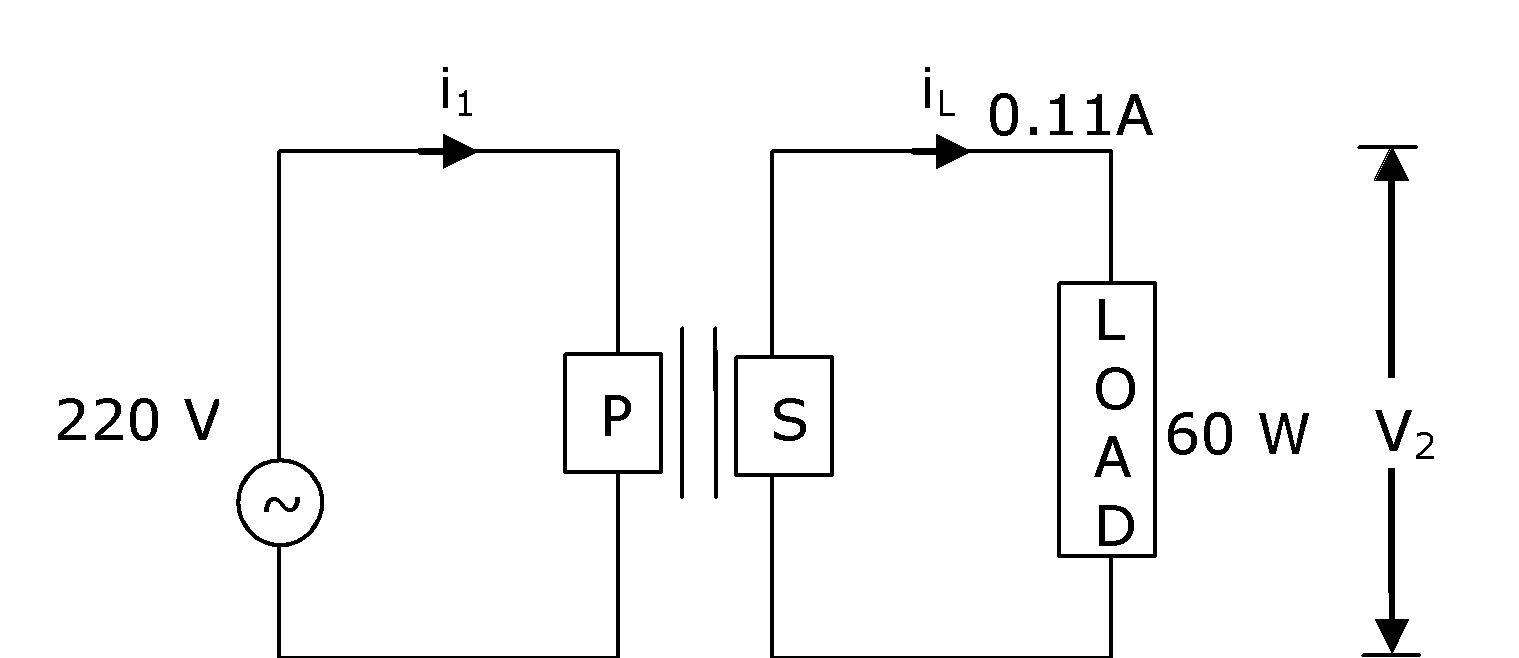1. a. Step down transformer
2. b. Auxiliary transformer
3. c. Step-up transformer
4. d. Autotransformer

Solution:

Ps = Vs × Is

60 = Vs × 0.11

Vs = 60/0.11 = 6000/11 = 545.45 volts, Vp = 220 volt

Vs > Vp so it is step up transformer

Question 8. Red light differs from blue light as they have:

1. a. Same frequencies and same wavelengths
2. b. Different frequencies and different wavelengths
3. c. Different frequencies and same wavelengths
4. d. Same frequencies and different wavelengths

Solution:

Red light and blue light have different wavelengths and different frequencies.

Question 9. The de-Broglie wavelength associated with an electron and a proton were calculated by accelerating them through the same potential of 100 V. What should nearly be the ratio of their wavelengths? (mp = 1.00727u; me = 0.00055u)

1. a. (1860)2 : 1
2. b. 43 : 1
3. c. 1860 : 1
4. d. 41.4 : 1

Solution:

$\lambda _{e}=\frac{12.27}{\sqrt{v}}$Å Or $\lambda =\frac{h}{\sqrt{2mqV}}$

$\lambda _{p}=\frac{0.286}{\sqrt{v}}$Å $\frac{\lambda _{e}}{\lambda _{p}}=\sqrt{\frac{m_{p}}{m_{e}}} = \sqrt{\frac{1.00727}{0.00055}}=\sqrt{1831}\simeq 43$

$\frac{\lambda _{e}}{\lambda _{p}}=\frac{12.27}{0.286}=43$

Question 10. A charge Q is moving $\vec{dl}$ distance in the magnetic field $\vec{B}$. Find the value of work done by $\vec{B}$.

1. a. Infinite
2. b. 1
3. c. –1
4. d. Zero

Solution:

$\vec{F}= q(\vec{V}\times \vec{B})$;

Since force F on a point charge by magnetic field $\vec{B}$ is always perpendicular to $\vec{V}$; W = 0

Question 11. Amplitude of a mass spring system, which is executing simple harmonic motion decreases with time. If mass = 500g, Decay constant = 20 g/s then how much time is required for the amplitude of the system to drop to half of its initial value?

(ln 2 = 0.693)

1. a. 15.01 s
2. b. 17.32 s
3. c. 0.034 s
4. d. 34.65 s

Solution:

$A = Ao e^{\frac{bt}{2m}}$

bt/2m = ln2 = 0.693

t = 2m/b × 0.693

t = 2 × 500/20 × 0.693

t = 50 × 0.693 = 34.6 sec.

Question 12. A resistor develops 500 J of thermal energy in 20s when a current of 1.5A is passed through it. If the current is increased from 1.5A to 3A, what will be the energy developed in the 20s.

1. a. 500 J
2. b. 1000 J
3. c. 2000 J
4. d. 1500 J

Solution:

I2RT= H

$\frac{H_{1}}{H_{2}} = \left ( \frac{I_{1}}{I_{2}} \right)^{2}$

$H_{2} = 500 \times \left ( \frac{3}{\frac{3}{2}} \right )^{2} = 2000 J$

Question 13. Calculate the value of mean free path (λ) for oxygen molecules at temperature 27°C and pressure 1.01 × 105 Pa. Assume the molecular diameter 0.3nm and the gas is ideal. (k = 1.38 × 10-23 jK-1)

1. a. 102 nm
2. b. 32 nm
3. c. 58 nm
4. d. 86 nm

Solution:

Given: P = 1.01 × 105 Pa

d = 0.3 nm

k = 1.38 × 10-23 Jk-1

T = 27°C

$\lambda _{mean} = \frac{KT}{\sqrt{2}\pi d^{2}p}$

$\frac{1.38\times 10^{-23}\times 300}{\sqrt{2}\times 3.14\times0.09 \times10^{-18} \times1.01\times 10^{5}}$

⇒ 102 nm

Question 14. A bimetallic strip consists of metals A and B. It is mounted rigidly as shown. The metal A has higher coefficient of expansion compared to that of metal B. When the bimetallic strip is placed in a cold bath, it will:1. a. Not bend but shrink
2. b. Neither bend nor shrink
3. c. Bend towards the right
4. d. Bend towards the left

Solution: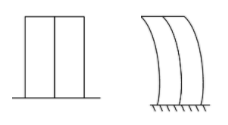αA > αB

Length of both strips will decrease

∆LA > ∆LB

⇒ A will be shorter and hence will bend towards the left.

Question 15. The following logic gate is equivalent to:1. a. NOR Gate
2. b. AND Gate
3. c. OR Gate
4. d. NAND Gate

Solution: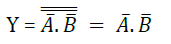So, given logic gates circuit is a NOR gate

OR

Truth table

 A B Y 0 0 1 0 1 1 1 0 1 1 1 0

Question 16. Two identical antennas mounted on identical towers are separated from each other by a distance of 45 km. What should nearly be the minimum height of the receiving antenna to receive the signals in line of sight? (Assume radius of earth is 6400 km)

1. a. 19.77 m
2. b. 79.1 m
3. c. 158.2 m
4. d. 39.55 m

Solution:

D = 2√2Rh

$H = \frac{D^{2}}{8R} = \frac{45^{2}}{8\times 6400}km = 39.55$

Question 17. The magnetic field in a region is given by $\vec{B} = B_{o}\left ( \frac{x}{a} \right )\hat{k}$. A square loop of side d is placed with its edges along the x and y axes. The loop is moved with a constant velocity $\vec{v} = v_{o}\hat{i}$. The emf induced in the loop is:1. a. $\frac{B_{o}v_{o}d^{2}}{2a}$
2. b. $\frac{B_{o}v_{o}d^{2}}{a}$
3. c. $\frac{B_{o}v_{o}d}{2a}$
4. d. $\frac{B_{o}v^{2}_{o}d}{2a}$

Solution: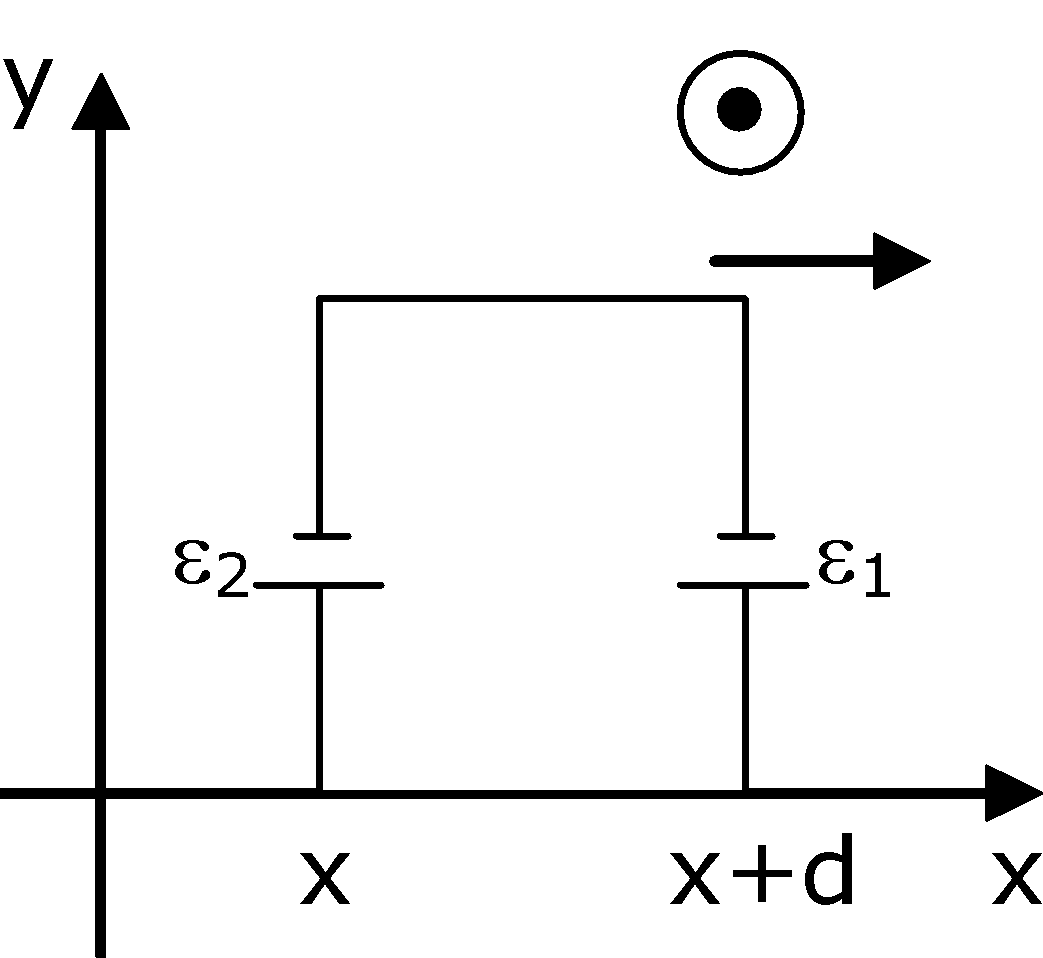$\varepsilon _{1} = \frac{B_{o}(x+d)}{a}v_{o}d$

$\varepsilon _{2} = \frac{B_{o}\: x}{a}v_{o}d$

net = ∈1 - ∈2

= $\frac{B_{o}v_{o}d^{2}}{a}$

Question 18. The half-life of Au198 is 2.7 days. The activity of 1.50 mg of Au198 if its atomic weight is 198 g mol-1 is (NA = 6 × 1023/mol).

1. a. 252 Ci
2. b. 357 Ci
3. c. 240 Ci
4. d. 535 Ci

Solution:

Initial activity = Ao = λNo

$=\frac{In2}{T_{\frac{1}{2}}}\times \frac{1.5\times 10^{-3}}{198}\times 6\times 10^{23}$

$=\frac{In2}{2.7\times 3600\times 24}\times \frac{1.5\times 10^{-3}}{198}\times \frac{6\times 10^{23}}{3.7\times 10^{-10}}Ci$

= 366 Ci

∴ Approximately the correct option is (b)

Question 19. In order to determine the Young's Modulus of a wire of radius 0.2 cm (measured using a scale of least count = 0.001 cm) and length 1m (measured using a scale of least count = 1 mm), a weight of mass 1 kg (measured using a scale of least count = 1 g) was hanged to get the elongation of 0.5 cm (measured using a scale of least count 0.001 cm). What will be the fractional error in the value of Young's Modulus determined by this experiment.

1. a. 9%
2. b. 1.4 %
3. c. 0.9%
4. d. 0.14%

Solution: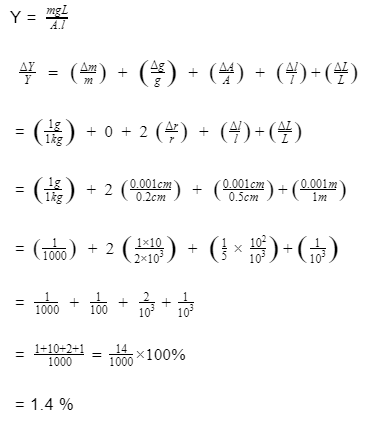Question 20. Find out the surface charge density at the intersection of point x = 3 m plane and x-axis in the region of uniform line charge of 8 nC/m lying along the z-axis in free space.

1. a. 47.88 C/m
2. b. 0.07 nC m-2
3. c. 0.424 nC m-2
4. d. 4.0 nC m-2

Solution: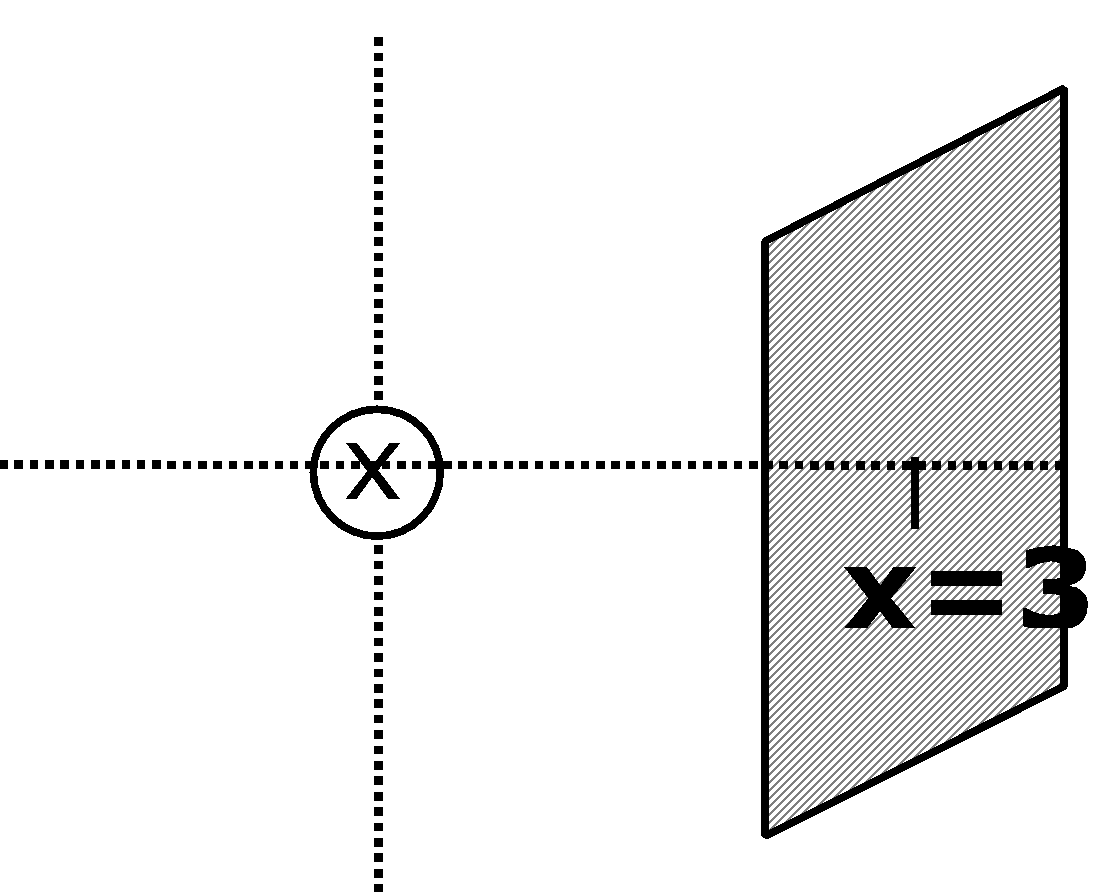Electric field due to wire is given by E = $\frac{2k\lambda}{r}$

Electric field with surface charge density E' = $\frac{\sigma}{\epsilon _{o}}$

$\frac{2k\lambda}{r} = \frac{\sigma}{\epsilon _{o}}$

$2\frac{1}{4\pi \epsilon _{o}}\frac{\lambda }{r} = \frac{\sigma}{\epsilon _{o}}$

$\frac{8\times 10^{-9}}{2\times 3.143} = \sigma$

σ = 0.424 n Cm-2

SECTION – B

Question 21. A closed organ pipe of length L and an open organ pipe contains gases of densities ρ1 and ρ2 respectively. The compressibility of gases are equal in both the pipes. Both the pipes are vibrating in their first overtone with the same frequency. The length of the open pipe is $\frac{x}{3}L \sqrt{\frac{\rho 1}{\rho 2}}$. where x is ______(Round off to the Nearest Integer)

Solution:

$V = \sqrt{\frac{B}{\rho}} = \sqrt{\frac{1}{k\rho }} (compressibility K = \frac{1}{B})$

for closed pipe f = $= \frac{3}{4L}\sqrt{\frac{1}{k_{1}\rho _{1}}}$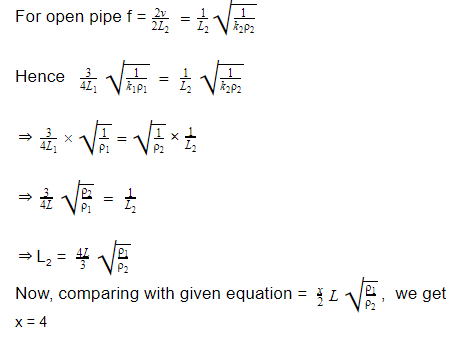Question 22. A deviation of 20 is produced in the yellow ray when the prism of crown and flint glass are chromatically combined. Taking dispersive powers of crown and flint glass as 0.02 and 0.03 respectively and refractive index for yellow light for these glasses are 1.5 and 1.6 respectively. The refracting angles for crown glass prism will be _______ ° (in degree). (Round off to the Nearest Integer)

Solution: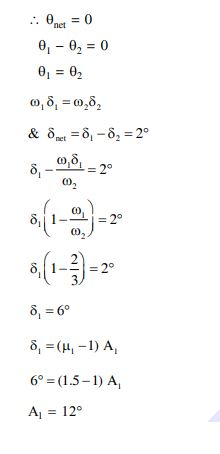Question 23. If one wants to remove all the mass of the earth to infinity in order to break it up completely. The amount of energy that needs to be supplied will be x/5 GM2 / R where x is __________. (Round off to the Nearest Integer). (M is the mass of earth, R is the radius of earth, G is the gravitational constant)

Solution:

Energy given = Uf – Ui

E = Uf – Ui

E = $0 - \left (\frac{3}{5}\frac{GM_{e}^{2}}{R_{e}} \right )=\frac{3}{5}\frac{GM_{e}^{2}}{R_{e}}$

So, x = 3

Question 24. In a parallel plate capacitor set up, the plate area of the capacitor is 2 m2 and the plates are separated by 1 m. If the space between the plates is filled with a dielectric material of thickness 0.5 m and area 2 m2 (see fig) the capacitance of the set-up will be ________∈o. (Dielectric constant of the material = 3.2) (Round off to the Nearest Integer)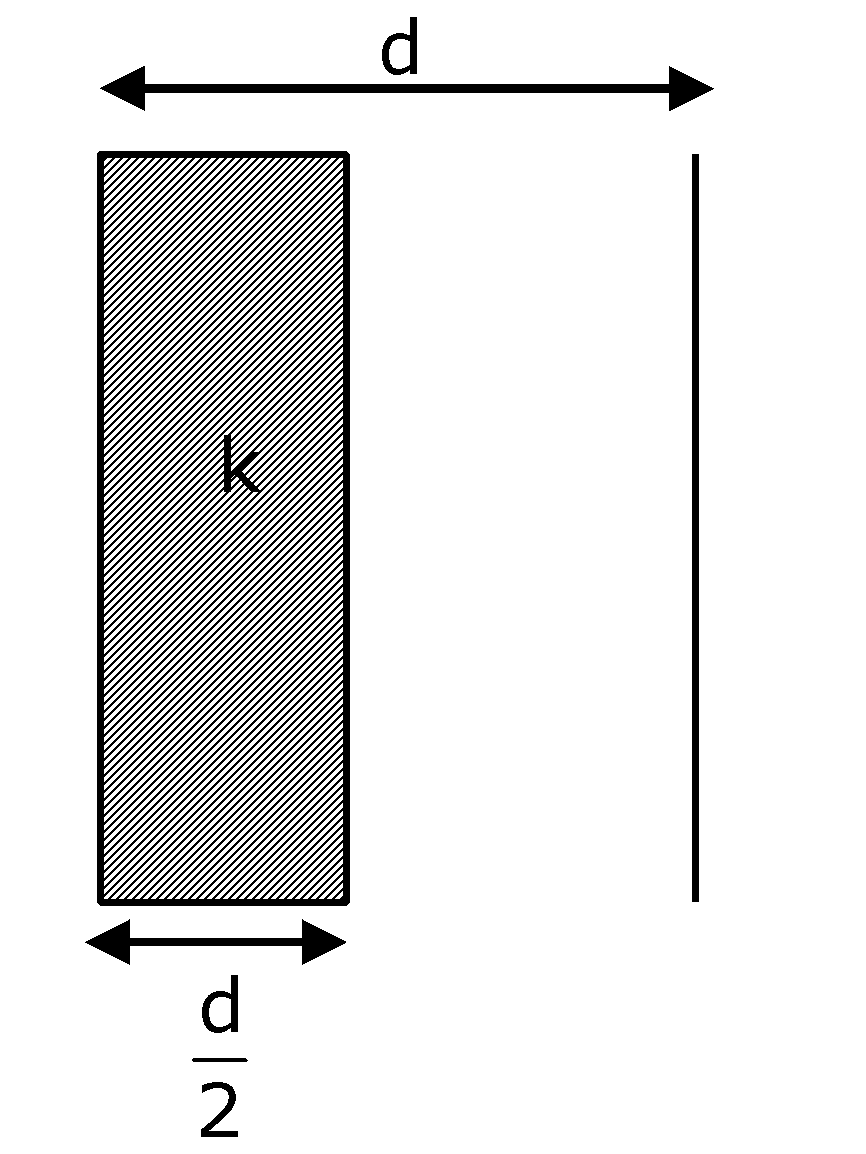Solution: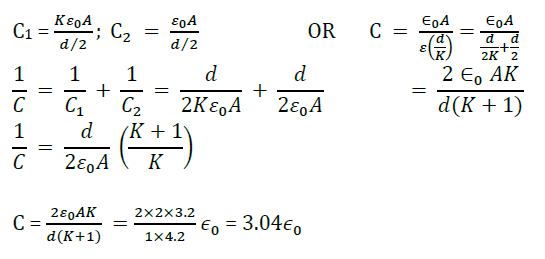Question 25. For an ideal heat engine, the temperature of the source is 127ºC. In order to have 60% efficiency the temperature of the sink should be _________ºC. (Round off to the Nearest Integer)

Solution:

η = (1 - T2 / T1) = 0.6 = 1 - T2 / 400

T2 / 400 = 0.4

T2 = 160 K

T2 = 160 – 273

T2 = -113°C

Question 26. A force $\vec{F} = 4\hat{i} + 3\hat{j} + 4\hat{k}$ is applied on an intersection point of x = 2 plane and x-axis. The magnitude of torque of this force about a point (2, 3, 4) is _______. (Round off to the Nearest Integer)

Solution:Given: $\vec{F} = 4\hat{i} + 3\hat{j} + 4\hat{k}$

$\vec{r}_{A} + \vec{r} = \vec{r}_{B}$

$\vec{r} = \vec{r}_{B}-\vec{r}_{A}$

$\vec{r} = (-3\hat{j} - 4\hat{k})$

$\left | \vec{\tau } \right | = \left |\vec{r}\times \vec{F} \right |$

$\vec{\tau } = \begin{vmatrix} \hat{i} & \hat{j} & \hat{k} \\ 0 & -3 &-4\\ 4 & 3 & 4 \end{vmatrix}$

$\vec{\tau } = -16\hat{j} - 12\hat{k}$

$\left | \vec{\tau } \right | = \sqrt{(-16)^{2}+(-12)^{2}} = \sqrt{400} = 20$

Question 27. The energy dissipated by a resistor is 10 ml in 1 s when an electric current of 2mA flows through it. The resistance is ________. (Round off to the Nearest Integer)

Solution:

H = i2Rt

10 × 10-3 = 4 × 10-6 R

∴ 10 × 103 / 4 = R

∴ R = 2500 Ω

Question 28. A swimmer can swim with a velocity of 12 km/h in still water. Water flowing in a river has a velocity of 6 km/h. The direction with respect to the direction of flow of river water he should swim in order to reach the point on the other bank just opposite to his starting point is ______°. (Round off to the Nearest Integer) (Find the angles in degrees)

Solution:Vm = 12 km/hr

Vr = 6 km/hr

Vm sin θ = vr

sin θ = 6/12

θ = 30°

α = 90 + 30

α = 120°

Question 29. A solid disc of radius 'a' and mass 'm' rolls down without slipping on an inclined plane making an angle θ with the horizontal. The acceleration of the disc will be 2/b g sin θ where b is _______ . (Round off to the Nearest Integer). (g = acceleration due to gravity; θ = angles as shown in figure)Solution:mg sin – f = macm .....(1) OR $a = \frac{gsin\theta }{1+\frac{k^{2}}{r^{2}}}$

f.a. = ma2 / 2.α .....(2) for disc = $\frac{k^{2}}{r^{2}} = \frac{1}{2}$

acm = α a .....(3) ⇒ $a = \frac{gsin\theta }{\frac{3}{2}} = \frac{2}{3}gsin\theta$

On solving,

acm = ⅔ g sin θ

⇒ b = 3

Question 30. A body of mass 2 kg moves under a force of $(2\hat{i}+3\hat{j}+5\hat{k})N$. It starts from rest and was at the origin initially. After 4s, its new coordinates are (8, b, 20). The value of b is _______. (Round off to the Nearest Integer)

Solution:

$(2\hat{i}+3\hat{j}+5\hat{k})N$

time = 4 sec

As the body starts from rest therefore position vector initially;

$\vec{r_{i}}(0\hat{i}+0\hat{j}+0\hat{k})$ and u (initial velocity) = 0

Given, $r_{f} = (x\hat{i}+y\hat{j}+z\hat{k})$

Now, from second equation of motion

$\vec{s} = \vec{u}t + \frac{1}{2}\vec{d}t^{2}$

$r_{f} - r_{i} = \frac{1}{2}\times \left ( \frac{2\hat{i}+3\hat{j}+5\hat{k}}{2} \right )\times (4)^{2}$

$(x\hat{i}+y\hat{j}+z\hat{k}) - (0\hat{i}+0\hat{j}+0\hat{k}) = 8\hat{i}+12\hat{j}+20\hat{k}$

$x\hat{i}+y\hat{j}+z\hat{k} = 8\hat{i}+12\hat{j}+20\hat{k}$

∴ The value of b = 12

### JEE Main 2021 Physics Paper March 16 Shift 2